Nuclear Physics NAT Level – 2

# Nuclear Physics NAT Level – 2 - Physics

Test Description

## 10 Questions MCQ Test Topic wise Tests for IIT JAM Physics - Nuclear Physics NAT Level – 2

Nuclear Physics NAT Level – 2 for Physics 2023 is part of Topic wise Tests for IIT JAM Physics preparation. The Nuclear Physics NAT Level – 2 questions and answers have been prepared according to the Physics exam syllabus.The Nuclear Physics NAT Level – 2 MCQs are made for Physics 2023 Exam. Find important definitions, questions, notes, meanings, examples, exercises, MCQs and online tests for Nuclear Physics NAT Level – 2 below.
Solutions of Nuclear Physics NAT Level – 2 questions in English are available as part of our Topic wise Tests for IIT JAM Physics for Physics & Nuclear Physics NAT Level – 2 solutions in Hindi for Topic wise Tests for IIT JAM Physics course. Download more important topics, notes, lectures and mock test series for Physics Exam by signing up for free. Attempt Nuclear Physics NAT Level – 2 | 10 questions in 45 minutes | Mock test for Physics preparation | Free important questions MCQ to study Topic wise Tests for IIT JAM Physics for Physics Exam | Download free PDF with solutions
 1 Crore+ students have signed up on EduRev. Have you?
*Answer can only contain numeric values
Nuclear Physics NAT Level – 2 - Question 1

### Graph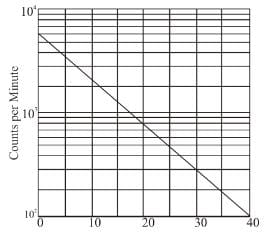A radioactive nucleus decays with the activity shown in the graph above. What is the half life of the nucleus? (in minutes) use log10 2 = 0.3 & log10e = 0.43

Detailed Solution for Nuclear Physics NAT Level – 2 - Question 1

Count at  t = 0 is 6 × 103
Count at  t  = 30 is 3 × 102
N0 = 6 × 103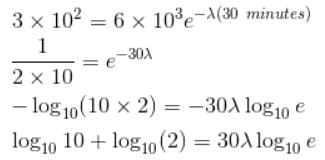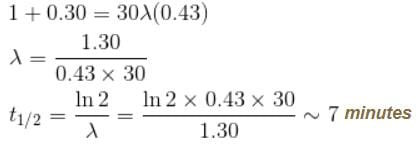*Answer can only contain numeric values
Nuclear Physics NAT Level – 2 - Question 2

### If the total angular momentum quantum number of a nucleus (A = 200) is J = 1, and if it is due to the rotation of the nucleus as a rigid body, find out the frequency. (in 1012 rad/s)

Detailed Solution for Nuclear Physics NAT Level – 2 - Question 2

A = 200
mass = 200 × 1.6 × 10–27 kg
J = 1
⇒  Angular momentum =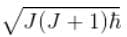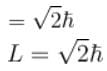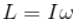(for a rigid body with moment of inertia = I)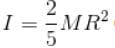(solid sphere since is A is quite high)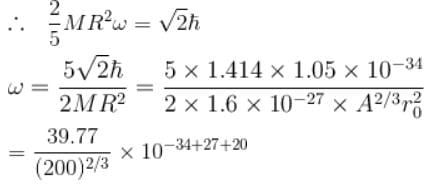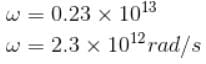*Answer can only contain numeric values
Nuclear Physics NAT Level – 2 - Question 3

### Plutonium 238Pu94 has an α decay half life of 90 years (2.7 × 109 s). If each of the α particles is emitted with 5.5 MeV energy, then find the average power released (till 50% has decayed) if there are 238 gms of 238Pu (i.e. 6 × 1023 atoms)(in Watts)

Detailed Solution for Nuclear Physics NAT Level – 2 - Question 3

Solution=

t1/2=2.7x109s

avg power=energy/time

=(No. of atoms decayed x 5.5MeV)/t1/2

=N0x5.5MeV/t1/2

=3x1023x5.5MeV/2.7x109

=(11.6x1023x10+16/109)x10-19

≈116W

*Answer can only contain numeric values
Nuclear Physics NAT Level – 2 - Question 4

If the energy of the α particle emitted by 231Am is 5.48 MeV, find the distance of closest approach between the α particle and 197Au nucleus (in 10–4 m)

Detailed Solution for Nuclear Physics NAT Level – 2 - Question 4

K.E, = 5.48 MeV
= 5.48 × 106 × 1.6 × 10-19 J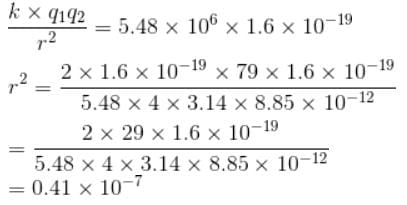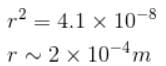*Answer can only contain numeric values
Nuclear Physics NAT Level – 2 - Question 5

Calculate the typical K.E. expected of an α particle confined within a nucleus if its emitted energy is 10 MeV. (Answer in MeV)

Assume the nuclear potential to be square will of potential V = –10 MeV.

Detailed Solution for Nuclear Physics NAT Level – 2 - Question 5

If the nuclear potential is a square well
From conservation of energy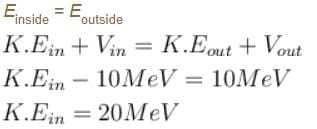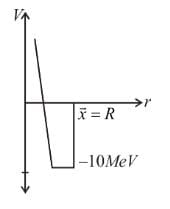*Answer can only contain numeric values
Nuclear Physics NAT Level – 2 - Question 6

Given that the nucleus density varies with r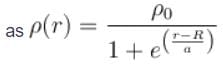where ρ0 = 0.14 nucleon/fm3,  R = 1.07 A1/3 and  a = 0.54  fm.

Find the surface thickness i.e. the distance between which density drops from 0.9ρ0 to 0.1ρ0 (in fm upto two decimal places)

Detailed Solution for Nuclear Physics NAT Level – 2 - Question 6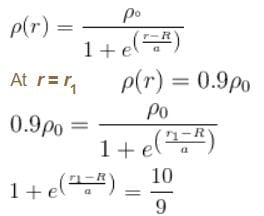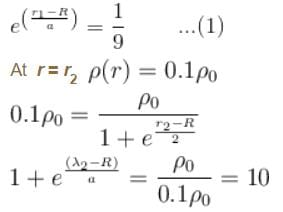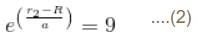Dividing (2) by (1)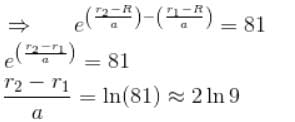Surface thickness = r2 – r1 = a × 4.39
r2 – r1 = 0.54 × 4.39
= 2.37 fm

*Answer can only contain numeric values
Nuclear Physics NAT Level – 2 - Question 7

The binding energy of a heavy nucleus is about 7 MeV/nucleon, whereas the B.E of a medium weight nucleus is about 8 MeV/nucleon. Therefore the total K.E liberated when a heavy nucleus undergoes symmetric fission is (in MeV).

Detailed Solution for Nuclear Physics NAT Level – 2 - Question 7

In case of symmetric fission the daughter nuclei have

Ad = A/2 each (daughter nuclei)
K.E. = diff. in B.E.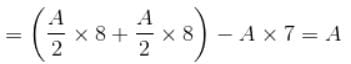For a heavy nucleus A ~ 200
K.E. ~ 200 MeV

*Answer can only contain numeric values
Nuclear Physics NAT Level – 2 - Question 8

What should be the order of the energy of the electron beam which is to be used to explore the nuclear charge distribution (take the radius to be of the order of 10 fm) and also nucleon charge distribution (r = 0.8 fm)(in GeV)

Detailed Solution for Nuclear Physics NAT Level – 2 - Question 8

For energy of electron,
λ ~ radius of the nucleus
= 10 fm = 10–14 m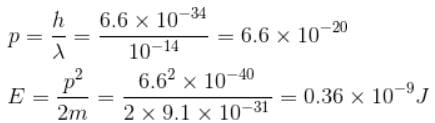= 0.19 × 1010eV ~ 2 × 109eV
~2 GeV

*Answer can only contain numeric values
Nuclear Physics NAT Level – 2 - Question 9

When α particles are directed onto atoms in a thin metal foil, some make very close collisions with the nuclei of the atom and are scattered at large angles. If an α particle with an initial kinetic energy of 5MeV happens to be scattered through an angle of 180°. Find the distance of closest approach to the scattering nucleus. (in fm)

Detailed Solution for Nuclear Physics NAT Level – 2 - Question 9

The particle obeys the coulomb's law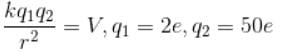(Helium)  (Silver)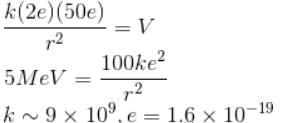In SI units r = 2.9 × 10-14m

*Answer can only contain numeric values
Nuclear Physics NAT Level – 2 - Question 10

A sample of radioactive nuclei of a certain element can decay only by γ emission and β emission. If half life for γ emission is 24 minutes and that for γ emission  is 36 minutes, the half life for the sample is how much? (in minutes)

Detailed Solution for Nuclear Physics NAT Level – 2 - Question 10

In such cases, the decay constant is the sum of the different decay constants.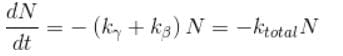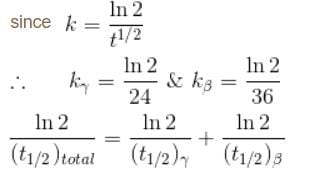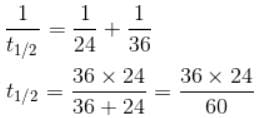t1/2 = 14.4 minutes(Scan QR code)